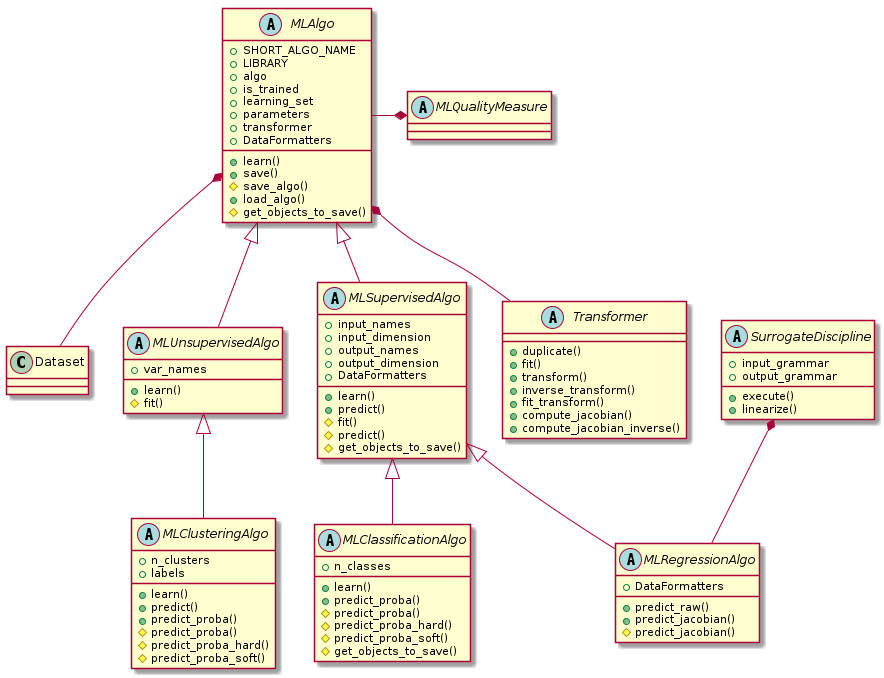# Introduction to machine learning¶

## Introduction¶

When an MDODiscipline is costly to evaluate, it can be replaced by a SurrogateDiscipline cheap to evaluate, e.g. linear model, Kriging, RBF regressor, … This SurrogateDiscipline is built from a few evaluations of this MDODiscipline. This learning phase commonly relies on a regression model calibrated by machine learning techniques. This is the reason why GEMSEO provides a machine learning package which includes the MLRegressionAlgo class implementing the concept of regression model. In addition, this machine learning package has a much broader set of features than regression: clustering, classification, dimension reduction, data scaling, …

Surrogate models

This module contains the base class for machine learning algorithms.

Machine learning is the art of building models from data, the latter being samples of properties of interest that can sometimes be sorted by group, such as inputs, outputs, categories, …

In the absence of such groups, the data can be analyzed through a study of commonalities, leading to plausible clusters. This is referred to as clustering, a branch of unsupervised learning dedicated to the detection of patterns in unlabeled data.

When data can be separated into at least two categories by a human, supervised learning can start with classification whose purpose is to model the relations between these categories and the properties of interest. Once trained, a classification model can predict the category corresponding to new property values.

When the distinction between inputs and outputs can be made among the data properties, another branch of supervised learning can be considered: regression modeling. Once trained, a regression model can predict the outputs corresponding to new inputs values.

The quality of a machine learning algorithm can be measured using a MLQualityMeasure either with respect to the learning dataset or to a test dataset or using resampling methods, such as K-folds or leave-one-out cross-validation techniques. The challenge is to avoid over-learning the learning data leading to a loss of generality. We often want to build models that are not too dataset-dependent. For that, we want to maximize both a learning quality and a generalization quality. In unsupervised learning, a quality measure can represent the robustness of clusters definition while in supervised learning, a quality measure can be interpreted as an error, whether it is a misclassification in the case of the classification algorithms or a prediction one in the case of the regression algorithms. This quality can often be improved by building machine learning models from standardized data in such a way that the data properties have the same order of magnitude.

Lastly, a machine learning algorithm often depends on hyperparameters to be carefully tuned in order to maximize the generalization power of the model.

class gemseo.mlearning.core.ml_algo.MLAlgo(data, transformer=mappingproxy({}), **parameters)[source]

An abstract machine learning algorithm.

Such a model is built from a training dataset, data transformation options and parameters. This abstract class defines the MLAlgo.learn(), MLAlgo.save() methods and the boolean property, MLAlgo.is_trained. It also offers a string representation for end users. Derived classes shall overload the MLAlgo.learn(), MLAlgo._save_algo() and MLAlgo._load_algo() methods.

Parameters:
• data (Dataset) – The learning dataset.

• transformer (TransformerType) –

The strategies to transform the variables. The values are instances of Transformer while the keys are the names of either the variables or the groups of variables, e.g. "inputs" or "outputs" in the case of the regression algorithms. If a group is specified, the Transformer will be applied to all the variables of this group. If IDENTITY, do not transform the variables.

By default it is set to {}.

• **parameters (MLAlgoParameterType) – The parameters of the machine learning algorithm.

Raises:

ValueError – When both the variable and the group it belongs to have a transformer.

class DataFormatters[source]

Decorators for the internal MLAlgo methods.

Noindex:

learn(samples=None, fit_transformers=True)[source]

Train the machine learning algorithm from the learning dataset.

Parameters:
• samples (Sequence[int] | None) – The indices of the learning samples. If None, use the whole learning dataset.

• fit_transformers (bool) –

Whether to fit the variable transformers.

By default it is set to True.

Return type:

None

Load a machine learning algorithm from a directory.

Parameters:

directory (str | Path) – The path to the directory where the machine learning algorithm is saved.

Return type:

None

save(directory=None, path='.', save_learning_set=False)[source]

Save the machine learning algorithm.

Parameters:
• directory (str | None) – The name of the directory to save the algorithm.

• path (str | Path) –

The path to parent directory where to create the directory.

By default it is set to “.”.

• save_learning_set (bool) –

Whether to save the learning set or get rid of it to lighten the saved files.

By default it is set to False.

Returns:

The path to the directory where the algorithm is saved.

Return type:

str

DEFAULT_TRANSFORMER: DefaultTransformerType = mappingproxy({})

The default transformer for the input and output data, if any.

IDENTITY: Final[DefaultTransformerType] = mappingproxy({})

A transformer leaving the input and output variables as they are.

LIBRARY: ClassVar[str] = ''

The name of the library of the wrapped machine learning algorithm.

SHORT_ALGO_NAME: ClassVar[str] = 'MLAlgo'

The short name of the machine learning algorithm, often an acronym.

Typically used for composite names, e.g. f"{algo.SHORT_ALGO_NAME}_{dataset.name}" or f"{algo.SHORT_ALGO_NAME}_{discipline.name}".

algo: Any

The interfaced machine learning algorithm.

property is_trained: bool

Return whether the algorithm is trained.

property learning_samples_indices: Sequence[int]

The indices of the learning samples used for the training.

learning_set: Dataset

The learning dataset.

parameters: dict[str, MLAlgoParameterType]

The parameters of the machine learning algorithm.

transformer: dict[str, Transformer]

The strategies to transform the variables, if any.

The values are instances of Transformer while the keys are the names of either the variables or the groups of variables, e.g. “inputs” or “outputs” in the case of the regression algorithms. If a group is specified, the Transformer will be applied to all the variables of this group.

## Development¶

This diagram shows the hierarchy of all machine learning algorithms, and where they interact with Dataset, MLQualityMeasure, Transformer and MLAlgoCalibration.